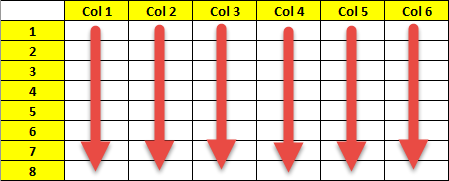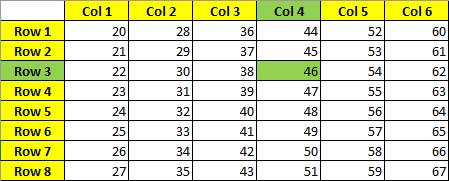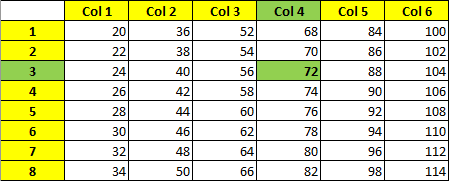# Storing arrays in computer memory [exercise]

I’ve got this interesting exercise to share with everyone. See if you can figure this out on your own first… Suppose a homogeneous array with 8 rows and 6 columns is stored in column major order starting at address 20 (base ten). If each entry in the array requires only one memory cell, what is the address of the entry in the third row and fourth column? What if each entry requires two memory cells? So, the problem #1 is following: Identify the address of the entry in the third row and fourth column of the array, in the given problem. And the problem #2 is to: Identify the address of the entry in the third row and fourth column of the array, in the given problem if each entry requires two memory cells.

# Solution

There is no need to use any coding language to solve this problem, as it can be very easily visualized.

Let’s visualize a homogeneous data structure (an array) that has 8 rows and 6 columns stored in column major order first. This is what it’ll look like:Now, let’s identify the address of the entry in the third row and fourth column of the array, taking in account that the array is starting at address 20 (base ten).

The address of the entry in the third row and fourth column = 46The preceding can be generalized to obtain a formula for converting references in terms of row and column positions into actual memory addresses. In particular, if we let c represent the number of columns in an array (which is the number of entries in each column), then the address of the entry in the i-th column and j-th row will be, as follows:

x + (c * (i – 1)) + (j – 1)

So in our case:

20 + (8 * (4-1))+(3-1)

20+8×3+3−1

20+24+3−1

Address [3,4] = 46

That is, we must move beyond i – 1 columns, each of which contains c entries, to reach the ith row and then j – 1 more entries to reach the jth entry in this row

## So how about, if each entry required two memory cells?

Let’s visualize this first.As we can see each entry is taking 2 memory cells, meaning that a 3rd row and 3th column is starting at address 72.

We can use the same formula we’ve found for a single memory cell array, but adjusting it, so each entry takes two memory cells to calculation:

x + 2 * (c * (i – 1)) + (j – 1)

So in our case:

20+2×(8×3+3−1)

20+2×(24+3−1)

20+2×26

20+52

Address [3,4] = 72

## Conclusion

Let’s conclude by saying, that the following formula:

x + m * (c * (i – 1)) + (j – 1)

x = starting memory address

m = number of memory cells each entry takes

c = number of entries in each column

i = calculate the entry in this column

j = calculate the entry in this row

; allows us to easily calculate address of any entry in any homogeneous array with any number of rows and any number of columns and starting at any base ten address, assuming that array is stored in a column major order.

Just for the reference:

If the array was stored in a row major order, generalized formula for converting references in terms of row and column positions into actual memory addresses would be very similar.

x + m * (c * (i – 1)) + (j – 1)

In the case of array stored in a row major order,  x is the starting address of the cell containing the entry in the first row and first column, c represent the number of columns in an array (which is the number of entries in each column), the i-th row and j-th column specifies the address of the entry to look up; and m represents the number of memory cells each entry takes.